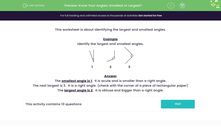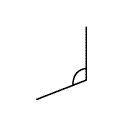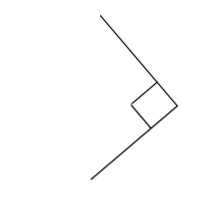# Know Your Angles: Compare Angle Sizes

In this worksheet, students will identify the largest and smallest angles from a choice of three.Key stage:  KS 2

Curriculum topic:   Geometry: Properties of Shapes

Curriculum subtopic:   Recognise Angles

Popular topics:   Angles worksheets, Geometry worksheets

Difficulty level:#### Worksheet Overview

This activity is about identifying the largest and smallest angles.

We can identify angles as follows: right angles (90 degrees), acute angles (smaller than a right angle), or obtuse angles (larger than a right angle).

Example

Identify the largest and smallest angles.1
 2
 3

The smallest angle is 1.  It is smaller than a right angle, so we call this an acute angle.

The largest angle is 2.  It is larger than a right angle, so we call this an obtuse angle.

Angle 3 is a right angle.

It is smaller than angle 2 but larger than angle 1.

We can spot a right angle by imagining the corner of a square or rectangle.

Also, right angles are often labelled with a square corner, like the one in this example.

Shall we have a go at some questions now?

### What is EdPlace?

We're your National Curriculum aligned online education content provider helping each child succeed in English, maths and science from year 1 to GCSE. With an EdPlace account you’ll be able to track and measure progress, helping each child achieve their best. We build confidence and attainment by personalising each child’s learning at a level that suits them.

Get started